Reach Us+44 1704 335730
Scheduling Microchps in a Group of Houses | OMICS International
Global Journal of Technology and Optimization
All submissions of the EM system will be redirected to Online Manuscript Submission System. Authors are requested to submit articles directly to Online Manuscript Submission System of respective journal.

# Scheduling Microchps in a Group of Houses

M.G.C. Bosman*, V.Bakker, A. Molderink, J.L. Hurink, G.J.M. Smit

University of Twente, Enschede, Netherlands

*Corresponding Author:
M.G.C. Bosman
University of Twente, Enschede, Netherlands
E-mail: [email protected]

Received date: October 2009; Revised date: November 2009; Accepted date: February 2010

Visit for more related articles at Global Journal of Technology and Optimization

#### Abstract

The increasing penetration of renewable energy sources, the demand for more energy efficient electricity production and the increase in distributed electricity generation causes a shift in the way electricity is produced and consumed. The downside of these changes in the electricity grid is that network stability and controllability becomes more difficult compared to the old situation. The new network has to accommodate various means of production, consumption and buffering and needs to offer control over the energy flows between these three elements. In order to offer such a control mechanism we need to know more about the individual aspects. In this paper we focus on the modelling of distributed production. Especially we look at the use of microCHP (Combined Heat and Power) appliances in a group of houses. The problem of planning the production runs of the microCHP is modelled via an ILP formulation both for a single house and for a group of houses.

#### Keywords

scheduling, micro-generation, model, household

#### Introduction

The increasing penetration of renewable energy sources, the demand for more energy efficient electricity production and the increase in distributed electricity generation causes a shift in the way electricity is produced and consumed  . Next to the production via large power plants, the consumers are producing more and more by themselves. Since uncontrolled distributed production can lead to unsatisfactory behaviour of the electricity network, distributed generation needs to be studied. Meanwhile the central production is changing due to higher efficiency and environmental demands. There is a growing demand for renewable energy sources, which also affects the existing electricity network. Stability of the electricity grid and controllability of the different ways of electricity generation are necessary elements in the new network .

In this paper we focus on the use of microCHP (Combined Heat and Power) as a distributed electricity producer. A microCHP appliance is a device that not only produces heat for in home use, just as a conventional boiler, but also electricity (see e.g. ). More generally, the model that is derived is meant for heat driven electricity generators on a household scale. Since there are different interested parties, e.g. the household, the electricity supplier and the grid operator, there are different objectives too. For this reason, the planning problem is split into two sub problems. The first sub problem is the heat driven control of the microCHP and concerns a single house. The second sub problem concerns the accumulated electricity generation and deals with a centrally controlled fleet of houses.

In the first sub problem we comply to a households' heat demand via a microCHP combined with a heat buffer. At each moment in time there must be enough heat stored in the buffer to supply the household demand. The problem is to find a good schedule in which the microCHP is switched on and off for a certain planning period, which in general is one day, using heat demand predictions to stay within the limits given by the heat buffer. A good schedule can be defined as a schedule in which electricity is produced at the most beneficial times for the household.

The second sub problem combines several single household problems and tries to make a schedule that is good for the fleet of houses. This means, that the fleet schedule has to comply to a prespecified production pattern, which is also based on electricity demand predictions. For example, the goal may be to deliver stability in the grid, i.e. the total production of the fleet should be stable over time.

The rest of the paper is organized as follows. In Section 2 the first sub problem is explained. Section 3 describes the modelling of a group of houses and in Section 4 some scenarios are given and discussed.

#### Single house model

In this section, we present a model for the use of a microCHP in a house and derive control methods from this setting. To achieve this, we need a model that represents a real house, including all kinds of residential behaviour and a realistic operation of the microCHP. The heat and electricity usage patterns of such a real house are called house dynamics. Residential behaviour, weather conditions (temperature, wind, sun, light/darkness), the opening of doors and windows, insulation and house dimensions are some of the parameters that influence the house dynamics. The exact influence of each of these parameters is unknown and hard to estimate. However, somehow we need to use these relations in predictions of domestic heat and electricity usage. In  an approach is presented to predict domestic heat demand by using neural networks. Household electricity prediction is discussed in e.g. .

We propose a discrete time model that incorporates the house dynamics and that gives a schedule for the runs of the microCHP appliance (periods the microCHP is producing). In this model we use time periods of fixed length. Within each time period the values of the decision variables are assumed to be fixed at the same value. The electricity and heat demand of all kinds of appliances in the house are aggregated over the time period, which gives one value for electricity and one value for heat demand for each time period. The advantage of a discrete model over a continuous model is that decisions must be made for time periods, not for moments. Also, in this way it is easier to take predictions (which are also given in time periods) into account. Moreover, a controller that controls the microCHP appliance uses discrete intervals too .

In the following sub sections we first present properties of the microCHP, which result in some constraints that influence the behaviour of runs of the microCHP. Next we describe the household demands and derive the scheduling problem for the microCHP from these demands. We use a heat buffer to create extra scheduling freedom, since it allows us to partly decouple heat production and heat consumption.

MicroCHP properties

The decision problem in a single house is to switch on or switch off a microCHP appliance. This decision has to be made for each time period j ∈T , where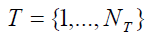denotes the set of time periods within the planning horizon. We denote this decision for time period j by a binary variable xj , which is 1 in case the microCHP is running and 0 in case it is not running.

in case the microCHP is running and 0 in case it is not running. Technical and economical reasons imply that for a good operation of the microCHP it needs to run for a minimum consecutive time in which it can at least reach the maximum power output. This minimum runtime MR is in general larger than one single time period, i.e.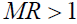(MR and other similar parameters are given in time periods). For this reason we need constraints that keep the microCHP running for consecutive time periods once it has started. The following two constraints take care of this: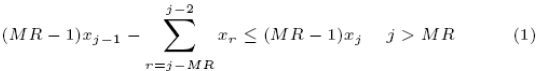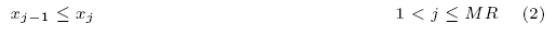Consider a time period j > MR. Constraint (1) states that, if the microCHP is not running in the previous period j −1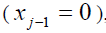the left hand side of the equation is smaller than or equal to 0, which means that the choice for the binary value of xj is free. On the other hand, if the microCHP is running during period j −1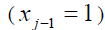, the choice for the value of xj is only free, if the microCHP has been running at least the MR time periods before period j . The left hand side of (1) is only 0, when the microCHP has been running during the period j −1 back to j − MR . If this is not the case, the left hand side of the equation is larger than 0, which fixes the choice for xj to 1.

Constraint (1) cannot be applied to the first MR time periods. For this reason, constraint (2) is introduced for the time periods 1 up to and including MR . It states that the value of xj is larger than or equal to the value of xj-1 . If the microCHP is started during the time periods 1,...,MR it stays running for the rest of these periods. As long as the microCHP has not been started, there is still full freedom in the choice of xj .

A second property of the microCHP is that it has to stay switched off at least a certain amount of time, once it has been stopped. This minimum offtime MO is required for the engine to prevent it from being stopped and immediately started too many times.

Since this constraint is somehow similar to the constraints regarding the minimum runtime MR , one may model this constraint similar as the minimum runtime constraints by just using variables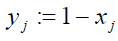. However, since in practice the minimum offtime MOis smaller than the minimum runtime MR , we can use a more tied formulation (more tied in the sense that the LP-relaxation of the ILP is more tied). The idea behind this formulation is explained below.

If we consider the change of the microCHP between two consecutive periods,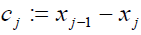the variables cj only take values from {−1,0,1} . Furthermore, if some cj= 1 , constraints (1) and (2) ensure that the previous MR −1 changes cj are zero. This allows us to model the minimum offtime constraints by: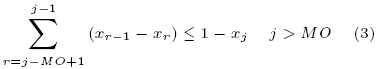Let us assume that the microCHP stopped after period t −1(i.e.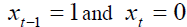), that the next time it has been started is period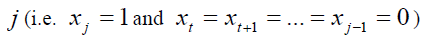and that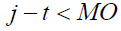In this case we get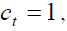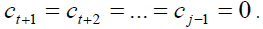As mentioned before, constraints (1) and (2) ensure that the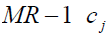values before t are all 0. Thus the left hand side of constraint (3) is 1 and the right hand side 0 for j , which is a contradiction. For all other situations for xj , constraint (3) does not lead to a restriction. Since a consecutive sequence ofvalues can contain at most one -1 and one +1 value, the only restrictive case is where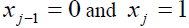. Thus, constraint (3) correctly models the minimum offtime constraint.

Constraints (1), (2) and (3) force the microCHP appliance to run correctly, i.e. the values that the sequence of decision variables xj can take correspond to the lengths of the periods the microCHP is running or not running in practice. However, there is no one-to-one relation between the running of the microCHP and the amount of heat and electricity that is produced. During starting and stopping less heat and electricity is produced than during normal operation. Therefore we need functions that give the relation between the run history of the microCHP and the corresponding heat/electricity production. For this reason we introduce the function g , which gives the value of the produced heat in period j (phj), based on the on/off status of the microCHP of period j ( xj ) and the produced heat in the previous period ( phj−1 ). Table 1 gives the heat production of the microCHP in all possible cases (MP denotes the maximum heat production of the microCHP), using 6 minutes time periods.

The produced electricity pej is coupled directly to the heat production. The amount of heat and electricity that is produced during the planning period is given by equations (4) and (5):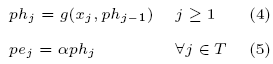where ph0 is set to 0.

All situations of the microCHP (starting, running at constant generation, stopping and not producing) are covered by the function g . The electricity production pej corresponds to the produced heat phj multiplied with a constant factor α .

Household demand

The constraints of the microCHP, mentioned in the previous sub section, are necessary constraints that must be fulfilled in order to have a correct functioning appliance. The question still remains when and for how long to run the machine. The decision to switch the microCHP on and off is mostly driven by the heat demand of the house, but the electricity demand may also be taken into account. Regarding the heat demand we have to ensure that the household is never getting out of supply. The electricity demand does not force the microCHP to be switched on or off at some moment in time, but it is important when considering the profit a household can make by producing at different times of the day (we use different prices over the day as in ). For this reason we incorporate the electricity demand in the objective function of the problem.

Both heat and electricity demand are assumed to be given via predictions. For a possible approach to get these predictions we refer e.g. to . The predictions are used as input data for the scheduling problem, which means that both heat and electricity consumption of a single house are considered to be fixed within the planning period of the scheduling problem. We introduce parameters CHj and CEj , representing the heat and electricity demand in time period j . At this point, we want to mention that in a real operation of the control method, besides the predictions also the concrete consumption has to be taken into account. However, the aim of this study is to get insight in the possibilities of controlling the microCHP. The achieved results may then be used as benchmarks for faster heuristics and runtime methods.

Next to a microCHP, we assume that the house uses a heat buffer. This heat buffer creates scheduling freedom for the switch on/off decisions for the microCHP, since it allows us to produce heat for a certain period j already in earlier time periods. For taking into account the buffer, we introduce a heat buffer level hj , which indicates how much thermal energy is in the buffer at the start of time period j . The change of this level is given by equations (6) and (7):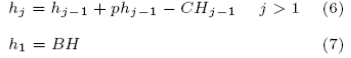where BH is the thermal energy that is available in the heat buffer at the begin of the first period.

The matching of electricity supply and demand is never a problem, since we assume that the electricity grid takes care of in house shortages and surpluses.

The relation between heat demand and heat supply is partly decoupled by using a heat buffer. In order to supply the given demand, the heat stored in the buffer is used. In order to always be able to supply future demand, we need to guarantee that there is a certain amount of heat extractable from the buffer during any period. For this reason we introduce a minimum heat level LL . We also introduce a maximum heat level UL , which is connected to the buffer capacity.

When the heat buffer level drops below the minimum level LL , the microCHP has to be switched on. The expectation is that the heat buffer soon contains no longer heat that can be extracted. Since we want to supply all heat demand, the microCHP then has to be switched on. The heat buffer has a certain capacity. This capacity cannot be exceeded. For this reason the maximum level UL is chosen, above which the microCHP needs to be switched off. When this level is used, the capacity is not expected to be exceeded (even when the appliance is still producing during the shutdown time). Constraints (8) and (9) take care of these two levels: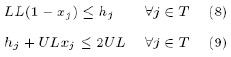In constraint (8), xj can take any value as long as hj is above LL . When hj is below LL , xj is forced to be 1. xj is forced to be 0 in constraint (9), when hj > UL . In the other case, xj is not restricted.

Resulting problem formulation

In the previous sub sections we modelled a single house that is equipped with a microCHP and a heat buffer. The runs of the microCHP are forced by the heat demand of the house together with the requirements of the heat buffer and the microCHP properties. The constraints more or less limit the total production and the number of runs. However, there is still space to decide on start times and run lengths. The decisions when to start and for how long to run are triggered via the objective function.

In general, the objective function depends on electricity production, in relation with in house parameters, as the heat and electricity demand, but also with parameters origining from outside the house, e.g. the prices on the electricity market. The objective function has the following general form: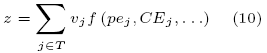If we choose the objective to be a linear function, we get an ILP formulation of the problem. This single house ILP formulation is the following: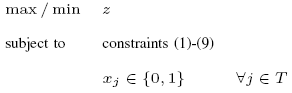When we choose 6 minutes as the length of each time period and set the planning horizon to 24 hours, we get 240 decision variables xj . Since there are some fixed relations (minimum runtime and minimum offtime) the decision problem for one house and for one day is relatively small. This would suggest another approach of the problem, rather than a costly ILP.

Dynamic programming

In this section we propose a dynamic programming formulation (DP) of the single house problem given in the previous sub section. The planning horizon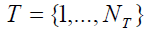and the decisions to switch the microCHP on/off or keep the state (running or off) unchanged, remain the same. However we do not actively use the decision variable xj anymore.

We introduce a state tuple (A, B,C) to describe the situation in a certain time period. A denotes the number of time periods that the state of the microCHP is unchanged until the start of the current period (positive values indicating that the microCHP is running and negative valu es indicating that the microCHP is off). B is the total number of periods the microCHP has been running for the whole planning period until the current period and C is the number of runs of the microCHP which have already been finished. For each time period j ∈T and state (A, B,C) we define the cost function Fj (A, B,C) , which aims at minimizing the costs from time period j until the end of the planning horizon, NT , assuming that the current situation is characterized by the state (A, B,C) . The costs between two consecutive states in sequential time periods can vary for different time periods and states. In this way the costs represent the objective function in Section 2.3. The hard constraints in Section 2.1 and 2.2 are represented by costs of ∞ .

Regarding the heat demand in Section 2.2 we have the following. The total amount of generated heat (electricity) can be deducted from the combination of A , B and C . Since the demand of each time period and the begin level of the heat buffer are known, we can deduce for each state in each time period whether lower and upper levels of the heat buffer are exceeded or not. In case there is a violation, a penalty of ∞ is given to the corresponding state change. The minimum runtime and offtime constraints from Section 2.1 now can be taken into account by looking at the value of A and penalizing `wrong' state changes in the DP with a value of ∞ .

In Figure 1 a representation of state (3,13,2) is given. Note that the exact start positions and lengths of completed runs are not taken into consideration via the state tuple (A, B,C) . Only the necessary information (the total amount of generated heat and the previous state change of the microCHP) is stored. The total amount of generated heat is enough information to fulfil the heat buffer constraints and the previous state change of the microCHP is enough information to fulfil the runtime/offtime constraints.

Figure 2 shows an example for two state changes that are possible from (3,13,2) . In the given case, switching the microCHP off is not possible, either due to minimum runtime constraints (MR > 3 ) or due to heat demand (in this case the lower level is reached at the start of time period j ).

Via a backtracking algorithm the value of F0(0,0,0) can be calculated. The path(s) corresponding to this value give the state tuple changes which correspond to the xj values in the ILP formulation. Since there are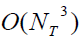state tuples in each period and there are NT time periods to evaluate, the dynamic programming approach of the single house model has runtime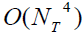.

For solving a single house problem the DP approach is surely preferable to the ILP approach. However, if we introduce several houses into a combined model, the state space explodes. Therefore for the problem of controlling a fleet of houses, we continue with our ILP formulation.

Model of a group of houses

For a single house, as modelled in Section 2, the problem is heat led and optimized for electricity production at beneficial times. When we consider a group of houses, the individual production is still based on the heat demand within the single house, since we do not consider sharing heat between houses, due to expected large energy losses. However, in the context of a Virual Power Plant (VPP) , we can start thinking of combining the individual production capacities of all houses into a concept of aggregated electricity production. On top of a heat led single house we place electricity led fleet production constraints in the model. This means that we do not only want to optimize for electricity production via soft objective variables, but that we use hard constraints on the total electricity production of the fleet of houses over all time periods. More precisely, we specify a production pattern in advance, that must be met, within some limits.

Electricity production

The production pattern can be derived from e.g. the day ahead market prices on the APX  or the load control objectives as in . This pattern gives the preferable amount of electricity to be produced in the periods. As in general it is not possible to precisely match this pattern, we incorporate this pattern in two different ways into the model. On the one hand we only allow a certain deviation from the given pattern per period (this allowed deviation may be dependent on the time period) and on the other hand we penalize the deviation in the objective function.

Formally, we introduce a set of houses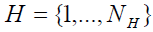. For each house i ∈ H we take the model from Section 2.3 (note that each variable/parameter gets a superscript i for house i ) and we add the following constraint: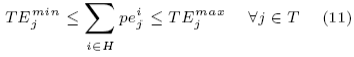in which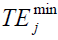and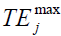represent the boundaries of the desired production pattern at time period j . The total produced electricity in all houses needs to be within these boundaries. The values ofanddetermine the deviation of the desired shape of the production pattern. If a feasible schedule is impossible, constraint (11) can be weakened by increasingand decreasing. If this is the case, the desired production pattern can still be incorporated in the objective function, such that the model still tries to map the schedule onto the intentional pattern.

Resulting problem formulation

The objective function has the following general form: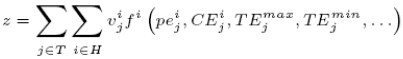Finally, the two sub problems combined result in the following formulation:

If we choose a linear function as the objective function we get an ILP formulation. For a problem, consisting of 10 houses and 240 periods (a schedule for one day), we have 2400 decision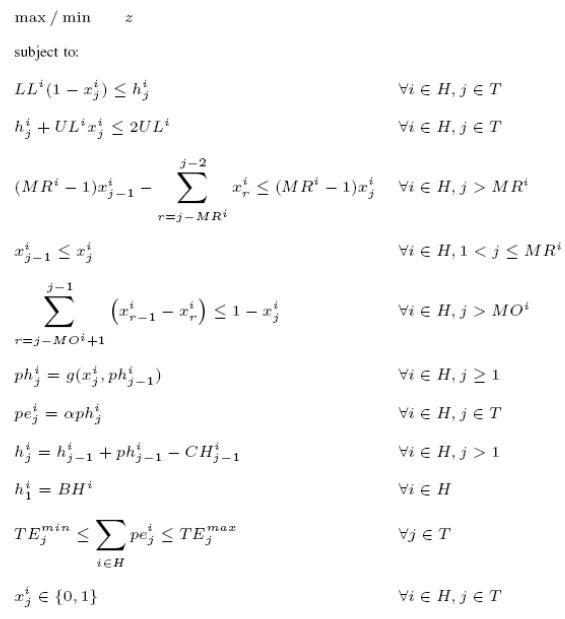variables xj Since we demand a certain amount of electricity production of all houses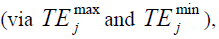local decisions in one house influence the decisions in other houses to switch on or off the microCHP. Unfortunately, this does not decrease the number of decisions which have to be made as easy as the dependencies in each house (minimum runtime/offtime) do.

#### Results and discussion

In Section 3 we propose a model of a group of houses, equipped with a microCHP appliance, and show an ILP formulation that combines the heat led single house model and the electricity led fleet production. This model can be used to derive feasible and possibly optimal schedules for the runs of the microCHP in all houses.

In this light, the model can play an important role in defining comparative benchmark scenarios to test future control methods in the context of a VPP. The schedules produced by the ILP solver can be used for an exploration of the possibilities and properties of using microCHP appliances in a fleet of houses.

To get more insight in the problem specific properties we define three scenarios. These scenarios are described in Section 4.1. Section 4.2 gives the schedules, which result from an implementation in AIMMS , and discusses the decisions that are made. Finally recommendations and future work are presented.

Scenarios

In our view, a future approach of controlling a fleet of microCHP appliances consists of an offline one-day-ahead schedule on the one side and online rescheduling methods during the operation of the houses on the other side. A first approach to the offline scheduling part is the model derived in this paper. For this reason we use a 24 hour planning period for scenarios to test our approach. As mentioned in Section 2, we use discrete time periods of 6 minutes, which results in 240 decision variables xj for each house.

The goal of the scenarios is to show that we can control the production of a fleet of houses in two ways. In the first scenario a constant preferable pattern is demanded, while all houses follow the same consumption pattern. The problem here is to investigate whether the production of individual houses can be shifted in time, since the production in the rest of the fleet limits the decision to let the microCHP run in each individual house. The second scenario tries to shift the production of houses to more beneficial periods in time. Meanwhile there is no restriction on the total production of the fleet. This scenario shows the capabilities of the fleet of houses to shift simultaneously runs in time. In the third scenario we combine the first two scenarios and use both the preferable production pattern and the objective function to steer the production runs.

Scenario 1

We consider a fleet of 10 houses. Since the first scenario is set up to see whether we can schedule runs of individual houses under fleet production constraints, we want to create a rather difficult situation. By increasing the heat demand of the houses we force many runs of the microCHPs. We use a heat demand of 350 Wh for all time periods. This value is the maximum heat demand we observed in the average heat profile derived from measurements in six houses for the durance of one week in winter . The total heat demand in such a house adds up to 84 kWh, which is about 1.6 times the average demand of the six houses. Each house uses the same microCHP with MR = 5 , MO = 5 , MP = 800Wh per six minutes and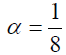, and a heat buffer with LL = 1 kWh and UL = 9 kWh. The heat level at the begin of the day varies per house, from 1 to 10 kWh with steps of 1 kWh. The electricity profile is a standard profile as described in . The preferable production pattern is bounded from above by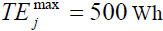for all time periods j . This indicates that a maximum of 5 microCHP appliances can run simultaneously at maximum production. The lower levelis set to 0, in order to allow some flexibility of the solution. Since the average fleet production needs to be about 0.44 kWh per time period, a feasible schedule needs to be tight to the upper levelThe objective is to match heat demand and supply with the minimum amount of production (so use the available heat in the heat buffer as much as possible).

Scenario 2

In the second scenario we again use a fleet of 10 houses, equipped with the same microCHP appliance and heat buffer as above. Also, the heat and electricity profile are the same as in the first scenario. The goal of this scenario is to show the flexibility of shifting runs in time, in a difficult setting in which a lot of heat production is required. We do not use a preferable production pattern, since we want to steer the production via the objective function. Based on the day ahead market prices of the APX , which are given in hours, we make a distinction of the benefit of the periods in 12 different types. The two hours with the lowest average electricity prices get the highest penalty costs attached; the two hours with the highest average electricity prices get the lowest penalty costs. The penalty costs have a logarithmic scale. Note, that we use penalty costs, since we consider a minimization of the objective.

Scenario 3

The third scenario combines the first and second scenario in the way that both the price based objective and the preferable production pattern are used. In this scenario we incorporated a normal heat profile instead of the maximum heat demand profile, since we want to show the flexibility of the model in a realistic scenario, in which there is more space to shift with runs. The objective function is the same as used in scenario 2.

Results

The schedules of the three scenarios are plotted in Figures 3, 4 and 5. Runs are plotted as blocks. The 10 houses are plotted on the y axis. The position of a block indicates the house the microCHP belongs to and the starting time of the run. The length of a block corresponds to the amount of time periods the microCHP is running subsequently. In case a background color is used, this color indicates the type of electricity price period, red corresponding to low prices and green to high prices. We implemented the developed model in AIMMS modelling software, using the CPLEX 11.1 solver.

Scenario 1

In Figure 3 we can see that at every time period no more than 5 houses are producing, which corresponds to constraint (11). To achieve this, a schedule is produced in which most of the runs are exactly the minimum runtime. Figure 6 shows the distribution of the run length of all runs in the first scenario. Using a minimum runtime of half an hour (5 periods) we can schedule a tight production pattern. The runtime in AIMMS is almost half an hour and it produces an almost optimal solution (the best solution used 995 production periods, while a lower bound gives 989.375 periods).

Scenario 2

The APX prices in Figure 4 show the beneficial production hours from red (not desired) to green (very beneficial). The hours in which production is not beneficial are almost not used for production. As can be seen in Figure 6 there are more longer runs than in the first scenario. These runs take place at the most beneficial hours. This schedule shows that we can decouple production from household demand to a large extent. The runtime in AIMMS is more than 2.6 hours and it produces a solution to the objective of 1.1332 ⋅1012 (which is a gap of 23 % to the lower bound of 8.6732 ⋅1011 ).

Scenario 3

The scenario that is proposed in the previous sub section is not solved by the CPLEX solver. However, when we stop using logarithmic penalty costs and steer more via the preferable production pattern, a feasible solution is given in Figure 5. In the hours with the highest prices, the peak of 5 microCHPs producing simultaneously is reached. The hours with the lowest price are not used for production. The schedule uses mostly short runs and gives a solution within half an hour, which is almost optimal (606 with a lower bound of 599.5875).

Conclusion

In this paper, we have shown that scheduling the microCHPs of a fleet of houses is possible. We can decouple production from consumption and we can influence the number of microCHP appliances that are producing at the same time, while we do not harm the households comfort.

In future work we want to use this insight to create controlling methods that are faster than the presented ILP approach. However, the quality of the schedules may not deviate too much from the best possible solution. Therefore, the ILP model can be used as a benchmark for new developed methods.

Besides considering the scheduling problem for a day ahead, we want to come up with online rescheduling methods, in order to incorporate real time electricity and heat consumption next to predictions.

#### References

Select your language of interest to view the total content in your interested language

### Article Usage

• Total views: 11909
• [From(publication date):
June-2010 - Nov 14, 2019]
• Breakdown by view type
• HTML page views : 8109Can't read the image? click here to refresh